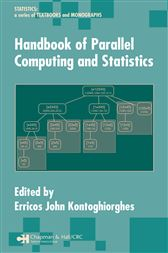Handbook of Parallel Computing and Statistics

Series: Statistics: A Series of Textbooks and MonographsCA\$71.13
Technological improvements continue to push back the frontier of processor speed in modern computers. Unfortunately, the computational intensity demanded by modern research problems grows even faster. Parallel computing has emerged as the most successful bridge to this computational gap, and many popular solutions have emerged based on its concepts, such as grid computing and massively parallel supercomputers. The Handbook of Parallel Computing and Statistics systematically applies the principles of parallel computing for solving increasingly complex problems in statistics research.

This unique reference weaves together the principles and theoretical models of parallel computing with the design, analysis, and application of algorithms for solving statistical problems. After a brief introduction to parallel computing, the book explores the architecture, programming, and computational aspects of parallel processing. Focus then turns to optimization methods followed by statistical applications. These applications include algorithms for predictive modeling, adaptive design, real-time estimation of higher-order moments and cumulants, data mining, econometrics, and Bayesian computation. Expert contributors summarize recent results and explore new directions in these areas.

Its intricate combination of theory and practical applications makes the Handbook of Parallel Computing and Statistics an ideal companion for helping solve the abundance of computation-intensive statistical problems arising in a variety of fields.

• CRC Press; December 2005
• ISBN: 9781420028683
• Edition: 1